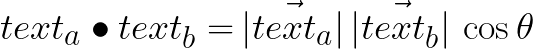# How to detect plagiarism in the text using Python

## Intro

Hi guys,

In this tutorial, we're going to learn how to Make a Plagiarism Detector in Python using machine learning techniques such as word2vec and cosine similarity in just a few lines of code.

## Overview

Once finished our plagiarism detector will be capable of loading a student’s assignment from files and then compute the similarity to determine if students copied each other.

## Requirements

To be able to follow through this tutorial you need to have scikit-learn installed on your machine.

### Installation

``````pip install -U scikit-learn
``````

### How do we analyze text?

We all know that computers can only understand 0s and 1s, and for us to perform some computation on textual data we need a way to convert the text into numbers.

### Word embedding

The process of converting the textual data into an array of numbers is generally known as word embedding.

The vectorization of textual data to vectors is not a random process instead it follows certain algorithms resulting in words being represented as a position in space. we going to use scikit-learn built-in features to do this.

### How do we detect similarity in documents?

Here we gonna use the basic concept of vector, dot product to determine how closely two texts are similar by computing the value of cosine similarity between vectors representations of student’s text assignments.Also, you need to have sample text documents on the student’s assignments which we gonna use in testing our model.

The text files need to be in the same directory with your script with an extension of .txt, If you wanna use sample textfiles I used for this tutorial download here

The project directory should look like this

``````.
├── app.py
├── fatma.txt
├── image.png
├── john.txt
└── juma.txt
``````

## Let's now build our Plagiarism detector

• Let’s first import all necessary modules
``````import os
from sklearn.feature_extraction.text import TfidfVectorizer
from sklearn.metrics.pairwise import cosine_similarity
``````

we gonna use OS Module in loading paths of textfiles and then TfidfVectorizer to perform word embedding on our textual data and cosine similarity to compute the plagiarism.

• Reading all text files using List Comprehension

We are going to use concepts of a list comprehension to load all the path textfiles on our project directory as shown below.

``````student_files = [doc for doc in os.listdir() if doc.endswith('.txt')]
``````
• Lambda function to Vectorize & Compute Similarity

We need to create two lambda functions, one to convert the text to arrays of numbers and the other one to compute the similarity between them.

``````vectorize = lambda Text: TfidfVectorizer().fit_transform(Text).toarray()
​
similarity = lambda doc1, doc2: cosine_similarity([doc1, doc2])
``````
• Vectorize the Textual Data

``````vectors = vectorize(student_notes)
​
s_vectors = list(zip(student_files, vectors))
``````
• Creating a Function to Compute Similarity

Below is the main function of our script responsible for managing the whole process of computing the similarity among students.

``````def check_plagiarism():
plagiarism_results = set()
global s_vectors
for student_a, text_vector_a in s_vectors:
new_vectors =s_vectors.copy()
current_index = new_vectors.index((student_a, text_vector_a))
del new_vectors[current_index]
for student_b , text_vector_b in new_vectors:
sim_score = similarity(text_vector_a, text_vector_b)
student_pair = sorted((student_a, student_b))
score = (student_pair, student_pair,sim_score)
return plagiarism_results
Let’s print plagiarism results
for data in check_plagiarism():
print(data)
``````
• Final code

When you compile down all the above concepts, you get the below full scripts ready to to detect plagiarism among student's assignments.

``````import os
from sklearn.feature_extraction.text import TfidfVectorizer
from sklearn.metrics.pairwise import cosine_similarity
​
student_files = [doc for doc in os.listdir() if doc.endswith('.txt')]
student_notes =[open(File).read() for File in  student_files]
​
vectorize = lambda Text: TfidfVectorizer().fit_transform(Text).toarray()
similarity = lambda doc1, doc2: cosine_similarity([doc1, doc2])
​
vectors = vectorize(student_notes)
s_vectors = list(zip(student_files, vectors))
​
def check_plagiarism():
plagiarism_results = set()
global s_vectors
for student_a, text_vector_a in s_vectors:
new_vectors =s_vectors.copy()
current_index = new_vectors.index((student_a, text_vector_a))
del new_vectors[current_index]
for student_b , text_vector_b in new_vectors:
sim_score = similarity(text_vector_a, text_vector_b)
student_pair = sorted((student_a, student_b))
score = (student_pair, student_pair,sim_score)
return plagiarism_results
​
for data in check_plagiarism():
print(data)
``````
• Output :

Once you run the above app.py the out will look as shown below

``````\$ python app.py
​
#__________RESULT ___________
('john.txt', 'juma.txt', 0.5465972177348937)
('fatma.txt', 'john.txt', 0.14806887549598566)
('fatma.txt', 'juma.txt', 0.18643448370323362)
``````

Congratulations you have just made your own Plagiarism Detector in Python, Now share it with your fellow peers, press Tweet now to share it.

In case of any comment, suggestion, or difficulties drop it in the comment box below and I will get back to you ASAP.

The original article can be found at kalebujordan.com